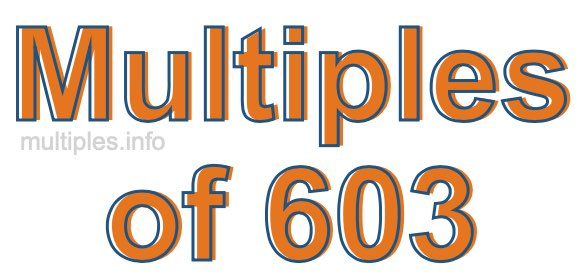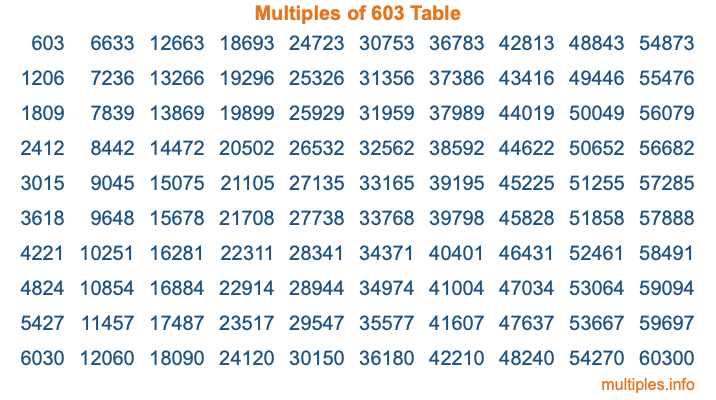Multiples of 603Welcome to the Multiples of 603 page. Here we will first teach you everything you will ever need to know about the multiples of 603, and then give you a study guide summary of everything we taught you to make sure you remember it all. Use this page to look up facts and learn information about the multiples of 603. This page will make you a multiples of six hundred three expert!

Definition of Multiples of 603
Multiples of 603 are all the numbers that when divided by 603 equal an integer. Each of the multiples of 603 are called a multiple. A multiple of 603 is created by multiplying 603 by an integer.

Therefore, to create a list of multiples of 603, you start with 1 multiplied by 603, then 2 multiplied by 603, then 3 multiplied by 603, and so on for as long as you want. Thus, the list of the first five multiples of 603 is 603, 1206, 1809, 2412, and 3015. To see a larger list of multiples of 603, see the printable image of Multiples of 603 further down on this page. We also have a category where you can choose any nth multiple of 603.

Multiples of 603 Checker
The Multiples of 603 Checker below checks to see if any number of your choice is a multiple of 603. In other words, it checks to see if there is any number (integer) that when multiplied by 603 will equal your number. To do that, we divide your number by 603. If the the quotient is an integer, then your number is a multiple of 603.

Is  a multiple of 603?

Least Common Multiple of 603 and ...
A Least Common Multiple (LCM) is the lowest multiple that two or more numbers have in common. This is also called the smallest common multiple or lowest common multiple and is useful to know when you are adding our subtracting fractions. Enter one or more numbers below (603 is already entered) to find the LCM.

Check out our LCM Calculator if you need more details about the Least Common Multiple or if you need the LCM for different numbers for adding and subtraction fractions.

nth Multiple of 603
As we stated above, 603 is the first multiple of 603, 1206 is the second multiple of 603, 1809 is the third multiple of 603, and so on. Enter a number below to find the nth multiple of 603.

th multiple of 603

Multiples of 603 vs Factors of 603
603 is a multiple of 603 and a factor of 603, but that is where the similarities end. All postive multiples of 603 are 603 or greater than 603. All positive factors of 603 are 603 or less than 603.

Below is the beginning list of multiples of 603 and the factors of 603 so you can compare:

Multiples of 603: 603, 1206, 1809, 2412, 3015, etc.

Factors of 603: 1, 3, 9, 67, 201, 603

As you can see, the multiples of 603 are all the numbers that you can divide by 603 to get a whole number. The factors of 603, on the other hand, are all the whole numbers that you can multiply by another whole number to get 603.

It's also interesting to note that if a number (x) is a factor of 603, then 603 will also be a multiple of that number (x).

Multiples of 603 vs Divisors of 603
The divisors of 603 are all the integers that 603 can be divided by evenly. Below is a list of the divisors of 603.

Divisors of 603: 1, 3, 9, 67, 201, 603

The interesting thing to note here is that if you take any multiple of 603 and divide it by a divisor of 603, you will see that the quotient is an integer.

Multiples of 603 Table
Below is an image of the first 100 multiples of 603 in a table. The table is in chronological order, column by column. The first column has the first ten multiples of 603, the second column has the next ten multiples of 603, and so on.The Multiples of 603 Table is also referred to as the 603 Times Table or Times Table of 603. You are welcome to print out our table for your studies.

Negative Multiples of 603
Although not often discussed or needed in math, it is worth mentioning that you can make a list of negative multiples of 603 by multiplying 603 by -1, then by -2, then by -3, and so on, to get the following list of negative multiples of 603:

-603, -1206, -1809, -2412, -3015, etc.

Multiples of 603 Summary
Below is a summary of important Multiples of 603 facts that we have discussed on this page. To retain the knowledge on this page, we recommend that you read through the summary and explain to yourself or a study partner why they hold true.

There are an infinite number of multiples of 603.

A multiple of 603 divided by 603 will equal a whole number.

603 divided by a factor of 603 equals a divisor of 603.

The nth multiple of 603 is n times 603.

The largest factor of 603 is equal to the first positive multiple of 603.

603 is a multiple of every factor of 603.

603 is a multiple of 603.

A multiple of 603 divided by a divisor of 603 equals an integer.

603 divided by a divisor of 603 equals a factor of 603.

Any integer times 603 will equal a multiple of 603.

Multiples of a Number
Here you can get the multiples of another number, all with the same attention to detail as we did for multiples of 603 on this page.

Multiples of
Multiples of 604
Did you find our page about multiples of six hundred three educational? Do you want more knowledge? Check out the multiples of the next number on our list!

Copyright  |   Privacy Policy  |   Disclaimer  |   Contact